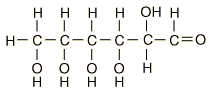# How do carbohydrates differ from hydrocarbons?

Jun 13, 2015

Carbohydrates contain $\text{C}$, $\text{H}$, and $\text{O}$. Hydrocarbons contain only $\text{C}$ and $\text{H}$.

#### Explanation:

The typical general formula for a hydrocarbon is ${C}_{n} {\text{H}}_{2 n + 2}$.

An example is hexane, ${\text{C"_6"H}}_{14}$.The typical general formula for a carbohydrate is ${C}_{n} {\text{H}}_{2 m} {O}_{m}$ or "C"_n("H"_2"O")_m, where n ≥ 3 and $m$ can be different from $n$.

The name carbohydrate comes from the formula "C"_n("H"_2"O")_m (carbon + hydrate).

A typical carbohydrate is glucose, ${\text{C"_6"H"_12"O}}_{6}$.All the polar $\text{OH}$ groups in carbohydrates tend to make them soluble in water.

Hydrocarbons are nonpolar and insoluble in water.